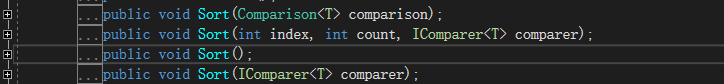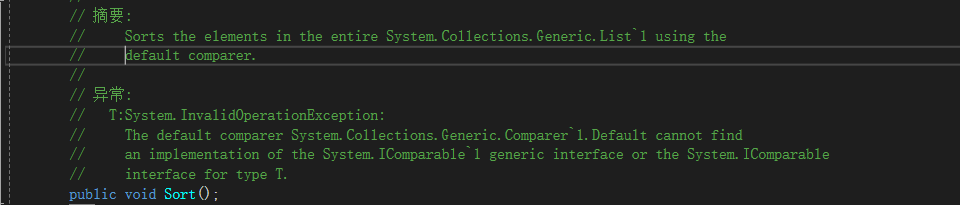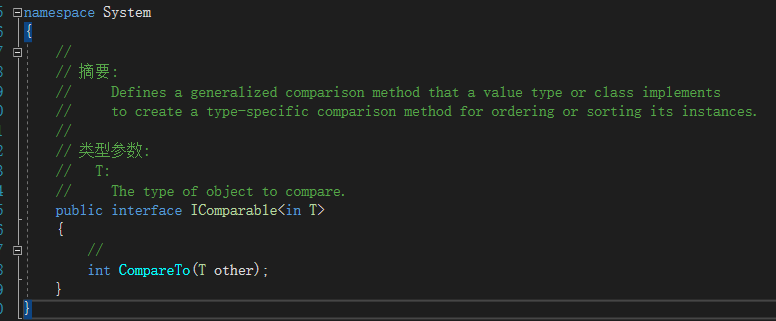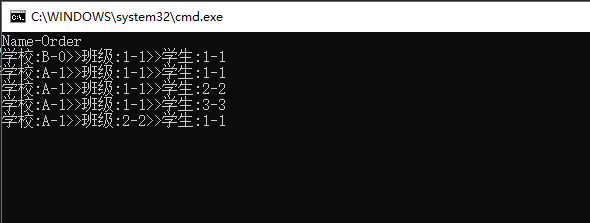# .Net集合排序的一种高级玩法实例教程

2018/5/28 1:33:48 人评论 次浏览 分类：C#

• 按学校编号（序号）排列
• 再按班级序号排列
• 再按学生学号排列

• 按学校名称排列
• 再按班级的名称排列
• 再按学生名称排列

```namespace Sort
{
public class School
{
public int? Order { get; set; }
public string Name { get; set; }
}

public class Class
{
public int? Order { get; set; }
public string Name { get; set; }
public School School { get; set; }
}

public class Student
{
public int? Order { get; set; }
public string Name { get; set; }
public Class Class { get; set; }

}
}```

《.Net中集合排序还可以这么玩》

Sort

.Net中，对List集合，有一个Sort字方法，让我们选中Sort方法，F12,看看Sort方法长哪样？IComparable接口值 含义 复数 该实例比传入的Other实例小。 0 该实例与传入的Other实例相等。 正数 该实例比传入的Other实例大。

• 按学校编号（序号）排列
• 再按班级序号排列
• 再按学生学号排列

• 按学校名称排列
• 再按班级的名称排列
• 再按学生名称排列

Int?类型(Nullable)和string已经实现了Compare方法，其中Nullable的如下：

```private int CompareInit(int? x, int? y)
{
if (x == null && y == null) //如果都是空 那么返回0相等
return 0;

if (x.HasValue && y == null) //如果传入X有值，但是Y是空的，那么X比Y小 返回-1。
return -1;

if (x == null && y.HasValue) //如果传入X为空，但是Y有值，那么X比Y大 返回1。
return 1;

if (x.Value > y.Value)
return 1;

if (x.Value < y.Value)
return -1;

return 0;   //否则两个数相等
}```

```public class Student : IComparable<Student>
{
public int? Order { get; set; }
public string Name { get; set; }
public Class Class { get; set; }
public int CompareTo(Student other)
{
if (ReferenceEquals(this, other)) return 0; //如果两个值的引用相同，那么直接返回相等。
if (ReferenceEquals(null, other)) return 1; //如果该实例是空的，但是传入的实例不是空的，那么返回1
//比较学校的序号
var compareResult = CompareInit(this.Class.School.Order, other.Class.School.Order);
if (compareResult != 0) return compareResult;
//比较班级的序号
compareResult = CompareInit(this.Class.Order, other.Class.Order);
if (compareResult != 0) return compareResult;
//比较学生的学号
compareResult = CompareInit(this.Order, other.Order);
if (compareResult != 0) return compareResult;
//如果以上还未区分出大小，比较学校的名称
compareResult = String.CompareOrdinal(this.Class.School.Name, other.Class.School.Name);
if (compareResult != 0) return compareResult;
//比较班级的名称
compareResult = String.CompareOrdinal(this.Class.Name, other.Class.Name);
if (compareResult != 0) return compareResult;
//比较学生的名称
return String.CompareOrdinal(this.Name, other.Name);

}```

```using System;
using System.Collections.Generic;

namespace Sort
{
class Program
{
static void Main(string[] args)
{
var students = InitData();
students.Sort(); //此处执行了Sort方法
Console.WriteLine("Name-Order");
foreach (var student in students)
{
Console.WriteLine(\$"学校:{student.Class.School.Name}-{student.Class.School.Order}>>班级:{student.Class.Name}-{student.Class.Order}>>学生:{student.Name}-{student.Order}");
}

}

static List<Student> InitData() //创建数据
{
var school1 = new School()
{
Order = 1,
Name = "A",

};
var school2 = new School
{
Name = "B",
Order = 0
};

var class1 = new Class
{
Order = 1,
Name = "1",
School = school1,
};

var class2 = new Class
{
Order = 2,
Name = "2",
School = school1,
};

var class3 = new Class
{
Order = 1,
Name = "1",
School = school2,
};

var student1 = new Student
{
Order = 1,
Name = "1",
Class = class1,
};

var student2 = new Student
{
Order = 2,
Name = "2",
Class = class1,
};

var student3 = new Student
{
Order = 3,
Name = "3",
Class = class1,
};

var student4 = new Student
{
Order = 1,
Name = "1",
Class = class2,
};

var student5 = new Student
{
Order = 1,
Name = "1",
Class = class3,
};
return new List<Student> { student5, student3, student4, student2, student1 };

}
}
}```https://github.com/liuzhenyulive/Sort (本地下载)

#### 您可能感兴趣的文章:

• .Net中的集合排序可以这么玩你知道吗
• 分享下Asp.Net面试题目及答案集合
• 关于.NET的集合总结
• ASP.NET4 GridView的四种排序样式详解
• asp.net下实现输入数字的冒泡排序

## 相关知识

• ### 简析 .NET Core 构成体系

前文介绍了.NET Core 在整个.NET 平台所处的地位，以及与.NET Framework的关系(原文链接)，本文将详细介绍.NET Core 框架的构成和各模块主要功能，以及如何实现跨平台。上图描述了 .NET Core的系统构成，最上层是应用层，是开发基于UI应用的框架集，包括了ASP.NET Core(用于…

2017/7/18 11:56:02